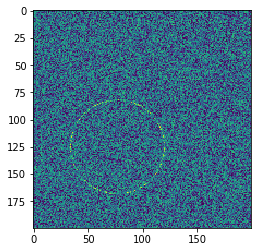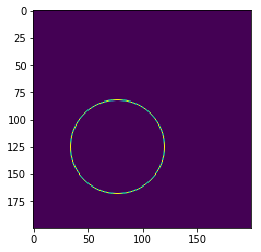top of page
Search

# Draw Noisy Circle In Machine Learning | Draw Circle Using Noise Area### Requirement:

The problem is to architect and train a model which is able to output the parameters of the circle present inside of a given image under the presence of noise. The model should output a circle parameterized by (row, column, radius) which specifies the center coordinates of the circle in the image and the radius of the circle. Please do not use a pre-trained architecture/model. (Excluding model training time)

### Example Images:

See attached

Deliverables: (All 3 required)

• Trained model and working find_circle method

• The standard output of the model training in a file called training output.txt to make sure that the training loss is visible in the output logs.

• The code used to define & train the model

Evaluation Criteria:

• Try to get as high of a score as you can but .9 is the minimum for your submission to consider using the metric AP@0.7. The provided main function will evaluate this metric in 1000 examples with a noise level of 2, we will use this main function to evaluate the model you produce.

• Model Architecture. You do not need to use an unnecessarily large CNN backbone (> 10 convolutional/linear layers or >1M trainable parameters)

• Loss function and optimizer used to train the model

• Code quality and cleanliness. Please make sure to follow the general python conventions

The attachments are only samples. You should generate your own data w/the function provided. Use main.py to generate the image and write a find_circle() function inside the main.py. You can use OpenCV or TensorFlow or PyTorch, or any other things that you need.

### import Libraries

```import numpy as np
from shapely.geometry.point import Point
from skimage.draw import circle_perimeter_aa
import matplotlib.pyplot as plt

```
```def draw_circle(img, row, col, rad):
rr, cc, val = circle_perimeter_aa(row, col, rad)
valid = (
(rr >= 0) &
(rr < img.shape) &
(cc >= 0) &
(cc < img.shape)
)
img[rr[valid], cc[valid]] = val[valid]

img = np.zeros((size, size), dtype=np.float)

# Circle
row = np.random.randint(size)
col = np.random.randint(size)

# Noise
img += noise * np.random.rand(*img.shape)

def find_circle(img):

# reshape input
data = img.reshape(1, -1)

# predict
prediction = model.predict(data)
prediction = prediction.reshape(3, 1)
return prediction

def iou(params0, params1):

return (
shape0.intersection(shape1).area /
shape0.union(shape1).area
)

def main():
results = []
for _ in range(1000):
print(_)
params, img = noisy_circle(200, 50, 2)
detected = find_circle(img)
results.append(iou(params, detected))
results = np.array(results)
print((results > 0.7).mean())
main()

```

```import numpy as np
from skimage.draw import circle_perimeter_aa

rr, cc, val = circle_perimeter_aa(row, col, rad)
valid = (
(rr >= 0) &
(rr < img.shape) &
(cc >= 0) &
(cc < img.shape)
)
img[rr[valid], cc[valid]] = val[valid]

img = np.zeros((size, size), dtype=np.float)

# Circle
row = np.random.randint(size)
col = np.random.randint(size)

# Noise
img += noise * np.random.rand(*img.shape)

```

build_model.py

```#import Libraries
import numpy as np
import matplotlib.pyplot as plt
import matplotlib
from circle import noisy_circle
from tensorflow.keras.models import Sequential
from tensorflow.keras.layers import Dense, Activation, Dropout
from tensorflow.keras.layers import Convolution2D, MaxPooling2D, Flatten

size = 200 # image size
bunch = 20000 # number of images

# containers for images and parameters
images = np.zeros((bunch, size, size), dtype=np.float)
circles = np.zeros((bunch, 3))

# generate bunch of images
for i in range(bunch):
circles[i], images[i] = noisy_circle(size, max_rad, 2)

# reshape and normalize the image data to mean 0 and std 1
X = (images.reshape(bunch, -1))# - np.mean(images)) / np.std(images)
print(f"\nReshaped image data: {X.shape}, {np.mean(X)}, {np.std(X)}")

# normalize circles' parameters to values between 0 and 1
y = circles.reshape(bunch, -1)# / size
print(f"Reshaped circles' parameters: {y.shape}, {np.mean(y)}, {np.std(y)}\n")

# split training and test sets
k = int(0.8 * bunch) # size of train set

# train
train_X = X[:k]
train_y = y[:k]

# test
test_X = X[k:]
test_y = y[k:]

# build model
model = Sequential()

# train model
model.fit(train_X, train_y, epochs=20, validation_data=(test_X, test_y), verbose=2)

# save model
model.save('circle.md')

# predict circle parameters on test images
pred_para = model.predict(test_X)

#pred_para = pred_y * size
pred_para = pred_para.reshape(len(pred_para), -1)

# plot an example
test_imgs = images[k:]
test_para = circles[k:]
k = 3

plt.figure(figsize=(10, 5))
plt.subplot(1, 2, 1)
plt.title("Original")
plt.imshow(test_imgs[k])

plt.subplot(1, 2, 2)
plt.title("Prediction")
plt.imshow(test_imgs[k])
plt.show()```

Output